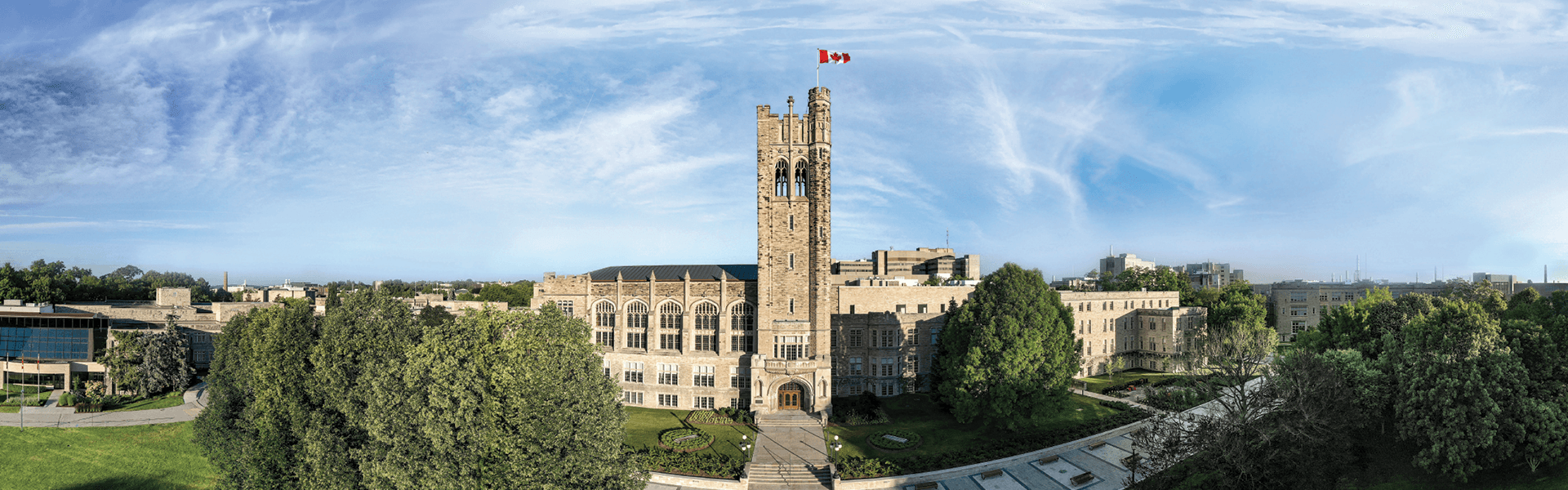# Course Outlines## Winter 2023

• AM 1201B: Calculus and Probability with Biological Applications
• AM 2811B: Linear Algebra II
• AM 2814G: Numerical Analysis
• AM 3813B: Nonlinear Ordinary Differential Equations and Chaos
• MATH 4958B: Special Topics in Mathematics (Covid Modelling)
• AM 4815B: Partial Differential Equations II
• CALC 1000B: Calculus I
• CALC 1301B: Calculus II
• CALC 1501B: Calculus II for Mathematical and Physical Sciences
• CALC 2503B: Advanced Calculus II
• MATH 1120B: Fundamental Concepts in Mathematics
• MATH 1225B: Methods of Calculus
• MATH 1228B: Methods of Finite Mathematics
• MATH 1229B: Methods of Matrix Algebra
• MATH 1600B: Linear Algebra I
• MATH 2120B: Intermediate Linear Algebra
• MATH 2122B: Real Analysis I
• MATH 2156B: Mathematical Structures II
• MATH 3120B: Group Theory
• MATH 3150B: Elementary Number Theory I
• MATH 4120B: Field Theory
• MATH 4152B: Algebraic Topology
• MATH 4156B: Complex Analysis II
• MATH 4959B: Special Topics in Mathematics (Commutative Algebra)

## Summer 2023

• AM 1201A: Calculus & Probability with Biological Applications
• CALC 1000A: Calculus I
• CALC 1301A: Calculus II
• CALC 1501A: Calculus II for Mathematical and Physical Science
• MATH 1228A: Methods of Finite Mathematics
• MATH 1229A: Methods of Matrix Algebra
• MATH 1600A: Linear Algebra I